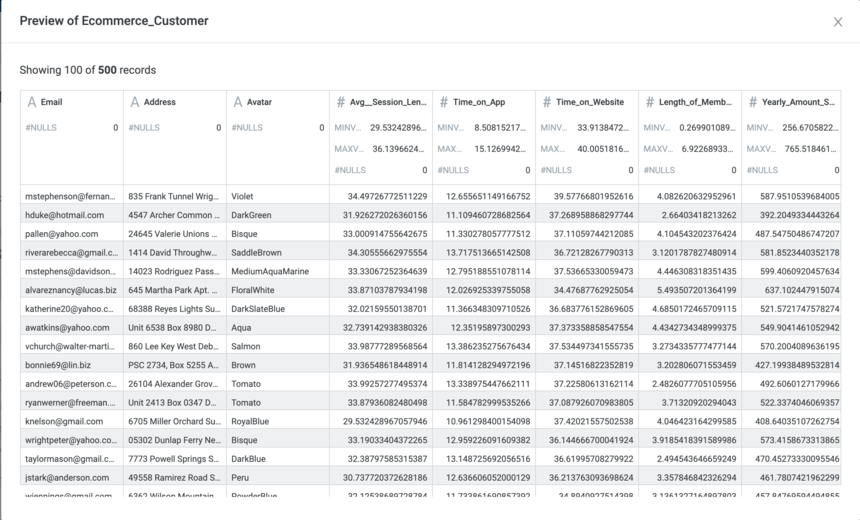2

[Data Science] Save Spark ML Model

Introduction

Incorta supports the Machine Learning(ML) model creation process by using Incorta Materialized Views (MV). While you can put the logic of applying the ML model testing and actually use of the ML model for inference in the same MV, the best practice is to separate them into different MVs.

This article shows how to save a model from one MV and use the saved model in another MV.

The sample data set we will use is eCommerce Customers.

There are eight columns:

• Email

• Avatar

• Avg. Session Length

• Time on App

• Time on Website

• Length of Membership

• Yearly Amount Spent.

This data set can be found on Kaggle

Assumption: eCommerce company based in New York City that sells clothing online but also has in-store style and clothing advice sessions. Customers come into the store, have sessions or meetings with a personal stylist, and then go home and order the clothes they want either on a mobile app or a website.

We need to predict 'Yearly Amount Spent.'

Here are the features or attributes collected in the dataset:

• 'Avg__Session_Length'

• 'Time_on_App'

• 'Time_on_Website'

• 'Length_of_Membership'Save Model

All the ML models should be saved to the same location on the disk in an on-premises environment.  A good place to create a folder is under <Incorta Tenant Folder>/data/models. Putting the model in the shared tenant folder will allow you to access it in a multiple-node environment.

Note: that for Incorta Cloud, you do not have the access the backend server, in order to get the tenant path, we can call the Spark property to get the path:

spark.conf.get("ml.incorta.tenant_path")

Different ML libraries may provide different ways of saving the models. Use the corresponding native ML library API to save the models. This article shows the ML Model Save functional from Spark ML.

You should create two MVs. One is Training for training your model, and the other one is Testing for testing the efficacy of your model. Save the model and use training data for evaluating the model in Training MV.

lr_model.write().overwrite().save("/incorta/IncortaAnalytics/Tenants/demo/data/models/Ecommerce_Customer_001")

You should load the saved model, use testing data for evaluating the model, and run the prediction in Testing MV. You need to consider Incremental in such a case.

Appendix

Training MV

# Takes a spark dataframe and pretty prints it
# Parameters:
# df: A spark dataframe
incorta.show(df)

# Takes a spark dataframe and pretty prints its count, mean, standard deviation, min, and max
# Parameters:
# df: A spark dataframe
incorta.describe(df)

x=df.columns
print(','.join(map(str, x)))

# VectorAssemblerTest

from pyspark.ml.linalg import Vectors
from pyspark.ml.feature import VectorAssembler

assembler = VectorAssembler(inputCols=['Avg__Session_Length', 'Time_on_App', 'Time_on_Website', 'Length_of_Membership'], outputCol='features')

#Split data into Training and Testing data sets

output = assembler.transform(df)
final_data = output.select('features', 'Yearly_Amount_Spent')
train_data,test_data = final_data.randomSplit([0.7,0.3])

# Train data

lr = LinearRegression(labelCol='Yearly_Amount_Spent')
lr_model = lr.fit(train_data)

print("Coefficients: " + str(lr_model.coefficients))
print("Intercept: " + str(lr_model.intercept))

#Save model

lr_model.write().overwrite().save("/incorta/IncortaAnalytics/Tenants/demo/data/models/Ecommerce_Customer_001")
trainingSummary = lr_model.summary

print("numIterations: %d" % trainingSummary.totalIterations)
print("objectiveHistory: %s" % str(trainingSummary.objectiveHistory))

trainingSummary.residuals.show()
print("RMSE: %f" % trainingSummary.rootMeanSquaredError)
print("r2: %f" % trainingSummary.r2)

# Test model

test_results = lr_model.evaluate(test_data)
test_results.residuals.show()
test_results.predictions.show()

# Root mean sqaured error

print("RMSE = %s" % test_results.rootMeanSquaredError)
print("R2 = %s" % test_results.r2)

#Prediction

unlabeled_data = test_data.select('features')
predictions = lr_model.transform(unlabeled_data)
predictions.show()

save(predictions)

Testing MV

#Prediction

unlabeled_data = df_testing.select('features')
unlabeled_data.show()

from pyspark.ml.regression import LinearRegressionModel
predictions = lr_model.transform(unlabeled_data)
predictions.show()

save(predictions)

By Suxin Ji, Data Engineering, Incorta Data Science & ML solution